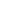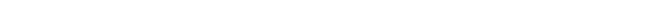## Discussion on magnetic and kinetic energy

When you are interested in physics you must read “Unbelievable“!

How is it be possible that the magnetic energy of an electron is the kinetic energy as well?

The answer to that question is that both forms of energy are different presentations of the same “dynamic” energy.

If an electron moves and collides with another particle the change in kinetic energy is transferred from one particle to the other. The kinetic energy of the electron changes, because the electron moves now with a different speed. The magnetic energy also has to change, because the charge of the electron now also moves with a changed velocity.

The same argument is valid when an electron (electric current) loses magnetic energy through magnetic induction. The electrons slows down and loses kinetic energy.

When we consider the formulas for magnetic energy of an electric current and the kinetic energy of a mass we find there are similarities. The magnetic energy of a current is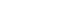[Joule], while the kinetic energy of a moving mass is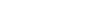[Joule].

Consider an electric current, where the electrons move twice as fast then the induced magnetic energy Wm will be four times as large and so is the kinetic energy of the moving electrons in the current. Both formulas, for the magnetic and kinetic energy, are consistent with the presentation of equivalence for kinetic and magnetic energy.

The logical consequence of the equivalence for magnetic and kinetic energy is, that every mass that moves possesses kinetic energy, also possesses magnetic energy!

The moving proton and magnetic energy
The proton is, like the electron, a single charged (+Qe) particle. The observable difference between the proton and electron is the opposite sign of the charge and the difference in mass. In chapter “The Proton and Neutron” we see that the intrinsic energy of the mass of a proton can be expressed the equation: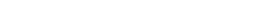Where the mass of a proton Mp is accounted the electrostatic energy for half the mass and for the other half magnetic spin energy. Rp is the radius of the bulb shaped proton.

The magnetic energy of the induced magnetic field (Wm) the moving proton will accordingly be: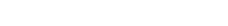The moving hydrogen atom and neutron
The proton is a positive charged particle. The moving proton must posses magnetic energy. But if kinetic energy is the same as magnetic energy, any moving mass must posses magnetic energy!

The hydrogen atom possesses no electric field outside the radius of the molecule. All electrostatic energy and therefore all magnetic/kinetic energy of the moving hydrogen atom concentrate in the atom, between electron and proton.

The charge of a separated moving electron and proton is not shielded, as it is in the hydrogen atom. The separated electron and proton therefore have a much larger range in which the electric fields are present and can interact with other charged particles when there is relative movement. The electrostatic field in the hydrogen atom, and therefore the kinetic/magnetic energy, is contained in the space between the proton and the electron.

For the moving hydrogen atom the same arguments as for the moving electron and proton are valid. The kinetic energy of the hydrogen atom is the induced magnetic energy the moving electrostatic field between proton and electron. Outside the hydrogen atom there is no electrostatic field or dielectric displacement, so outside the atom there is no magnetic field or energy. The magnetic/kinetic energy is confined to the area of the electric field, between proton and electron, in the hydrogen atom.

When a proton and an electron fuse to a neutron, during the fusion process the potential electrostatic energy of proton and electron is transferred to kinetic energy (magnetic energy). Although fused, the positive and negative charges of proton and electron still oscillate in the neutron, so the electrostatic field between both charges still exists, only now concentrated and therefore confined in the neutron.

The neutron does not posses an electrostatic field we can observe, because the oscillation frequency of the neutron is far too high (approx. 2.10^26 Hz chapter “The Proton and Neutron”) to be detected. Not being detectable does not mean that the equivalence of magnetic and kinetic energy for a neutron would not be valid.

When J.J. Thomson (1881) derived that the EM-theory could not explain the electromagnetic mass of an electron, during which he brutally violated the energy conservation law, no one questioned the correctness of his conclusions. His false analysis was completely copied QM and served them as proof that the EM-theory was inadequate to describe elementary particles. Feynman copied Thomson’s mistake in his Lectures on Physics to which article I refer above.

Why did QM-physicists never question Thomson’s fundamentally false derivation?

The answer to this question is to my knowledge the relentless faith of physicists in Maxwell’ equations. Maxwell’s equations describe the interrelationship between the electric field, the magnetic field, the electric charge, and electric current. Although Maxwell’s equations are mathematically correct, apparently no physicist ever verified whether these equations are also valid in physical sense!

Apparently QM-physics from the end of the 19th century until today is nothing more than math. As long as derived mathematical equations look nice and seem intuitively not to violate fundamental physics laws they are accepted as physically correct. At least one can say that QM handles “theories” over the last century in an improper scientific way.

Nowadays many (top-) scientists know that QM incorrectly disqualified the EM-theory as being inadequate to describe elementary particles. Actually now they know the QM-approach is the fundamentally flawed theory.

Knowing this these scientists should, if they were scientists living up to the moral and ethical standards of their profession, address the mistakes. They decide however that their personal interest is of more importance than the moral and ethical standards of their profession.

Next chapter: The photonAutomated page speed optimizations for fast site performance